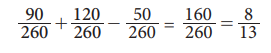Home | | Statistics 11th std | Addition Theorem of Probability

## Chapter: 11th Statistics : Chapter 8 : Elementary Probability Theory

Theorem : (Addition Theorem of Probability for Two Events)

## Theorem 8.4 : (Addition Theorem of Probability for Two Events)

If A and B are any two events in a random experiment, then

P(AŌł¬ B) = P(A) + P(B) ŌĆō P(AŌł®B)

### Proof:For any two events A and B, the shaded region in fig. 8.9 represents the event A Ōł¬ B.

AŌł¬ B =AŌł¬(B-(AŌł®B))

The events A and B-(A Ōł®B) are mutually exclusive.

Using Axiom 3Ōł¬

P(AŌł¬B) = P(A Ōł¬[B-(A Ōł®B)])

= P(A) + P[B-(AŌł®B)]

Since(AŌł®B)ŌŖéB,,

B = (A+B)Ōł¬(B ŌĆō(A+B))

The events on the right hand side are disjoints. Hence by axiom 3

P(B) = P ( A Ōł®B) + P( BŌĆō(AŌł®B))

i.e. P[BŌĆō(AŌł®B)] = P(B) ŌĆō P(AŌł®B) ŌĆ”ŌĆ”ŌĆ”ŌĆ”..(8.2)

Substituting (8.2) in (8.1)Ōł¬ it follows that

P(AŌł¬B) = P(A) + P(B) ŌĆō P(AŌł®B).

Corollary: If AŌł¬ B and C are any three events, then

P(AŌł¬ B Ōł¬C) = P(A) + P(B) + P(C) ŌĆō P(AŌł® B) ŌĆō P(AŌł® C) ŌĆō P(BŌł® C) + P(AŌł®BŌł®C)

### Example 8.12

In the Annual sports meet, among the 260 students in XI standard in the school, 90 participated in Kabadi, 120 participated in Hockey, and 50 participated in Kabadi and Hockey. A Student is selected at random. Find the probability that the student participated in (i) Either Kabadi or Hockey, (ii) Neither of the two tournaments, (iii) Hockey only, (iv) Kabadi only, (v) Exactly one of the tournaments.

### Solution:

n(s)=260

Let A : the event that the student participated in Kabadi

B : the event that the student participated in Hockey.

n(A) = 90; n(B) = 120; n(AŌł¬B) = 50(i) The probability that the student participated in either Kabadi or Hockey is

P (AŌł¬B) = P(A) + P(B) - P (AŌł®B)(ii) The probability that the student participated in neither of the two tournaments in(iii) The probability that the student participated in Hockey only is(iv) The probability that the student participated in Kabadi only(v) The probability that the student participated in exactly one of the tournaments isStudy Material, Lecturing Notes, Assignment, Reference, Wiki description explanation, brief detail
11th Statistics : Chapter 8 : Elementary Probability Theory : Addition Theorem of Probability |